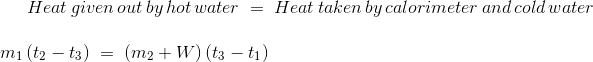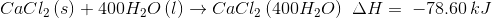Thermochemistry

# Objective

To determine:

• Water equivalent of calorimeter.
• Enthalpy of dissolution of given salt.

# The Theory

Thermochemistry is the study of heat and energy associated with a chemical reaction or a physical transformation.  A reaction may absorb or release energy. The reaction which absorbs energy in the form of heat is called endothermic reaction and that which releases energy in the form of heat is called exothermic reaction.

Measurement of heat changes are carried out in vessels called calorimeters. Metallic calorimeters are not used for measuring thermochemical changes because metals may react with substances. Stainless steel or gold plated copper calorimeters may be used. Reactions may also be carried out in beakers placed in a thermo flask, in a thermally insulated box or in a styrofoam cup. During measurement of heat changes, the calorimeter also absorbs some heat; this amount of heat should also be known. It is called calorimeter constant or water equivalent of calorimeter. To determine the water equivalent of calorimeter, known volume of hot water at a specified temperature is added to known volume of water contained in the calorimeter at room temperature. Since energy is conserved, the heat taken by calorimeter and cold water should be equal to heat given by hot water. Thus, we can write the following equation,​​It is known that when a solute is dissolved in a solvent, heat is absorbed or evolved. Thus, dissolution of a solute in a solvent is accompanied by enthalpy change (∆H) of the system. If heat is absorbed, ∆H is positive. If heat is evolved, ∆H is negative.

The enthalpy change per mole of a solute dissolved varies with the concentration of the solution. The enthalpy of dissolution is defined as the enthalpy change per mole of a solute when it is dissolved in a pure solvent to give a solution of specified concentration.

For example, when one mole of anhydrous calcium chloride is dissolved in 400 moles of water, 78.60 kJ heat is evolved. The thermochemical equation is written as:Similarly, when one mole of hydrated calcium chloride is dissolved in 400 moles of water, 18.83 kJ heat is absorbed. The thermochemical equation is written as:# Learning Outcomes

• Students understand the terms, water equivalent of calorimeter, enthaply of dissolution of salt etc.
• Students acquire the skill to perform the experiment in the real lab, once they understand the different steps.

Cite this Simulator: